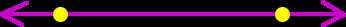# Online Math Dictionary: L

Easy to understand math definitions for K-Algebra mathematics
Just scroll down or click on the word you want and I'll scroll down for you!

 least common multiple LCM legs of a right triangle less than line linear equation linear inequality logarithm

Least Common Multiple (LCM)
The least common multiple is the smallest number that two (or more) given numbers both divide into.
Examples:  The LCM of 6 and 9 is 18.   The LCM of 4 and5 is 20.   The LCM of 2 and 10 is 10.
A common way to write it is LCM ( 69 ) = 18.
When used during fraction problems, another name for the least common multiple is the "least common denominator" (LCD).Legs of a right triangleThe legs of a right triangle are the two sides that are not the hypotenuse.   The legs are the two sides that are on either side of the right (90 degree) angle.Less Than
One number is less than another number when that number's value is smaller.
Example:   6 is less than 10.  In math, we write this as   6 < 10.LineA line is officially defined by the two points that it passes through.For more info on lines, check out one of the following lessons: For  graphing - Graphing Lines  How to find the slope of a line - Slopes How to find the equation of a line given two points. The equations of parallel and perpendicular lines.